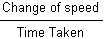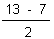HOME MATH DICTIONARY DOWNLOAD FEEDBACK DISCLAIMER
 Question: What is Acceleration ? Answer: Acceleration is the rate at which speed changes in a given direction. Acceleration is a vector quantity which is defined as the rate at which an object changes its velocity. i.eWhen speed is measured in metres per second and time in seconds, acceleration is in (metres per second) per second, usually written as: Metres per second2 or m/s2 or m s-2 For E.g., if the speed of a car traveling along a straight road increases steadily from 7 metres per second to 13 metres per second in 2 seconds, then the acceleration of the car in the direction of the road is== 3 m s-2 When a heavy object falls under gravity, its speed increases by about 10 m s-1 every second, so it has an acceleration of 10 m s-2 downwards.Acceleration, like velocity, is a vector quantity. Acceleration is Expressed as the first derivative of velocity with respect to time (that is, the rate of change of velocity), or equivalently as the second derivative of position. It is a vector quantity with dimension L T-2. In SI units, acceleration is measured in metres per second squared (m/s2). In common speech, the term acceleration is only used for an increase in speed (the magnitude of velocity); a decrease in speed is called deceleration. In physics, any increase or decrease in speed is referred to as acceleration, and also a change in the direction of velocity is an acceleration.